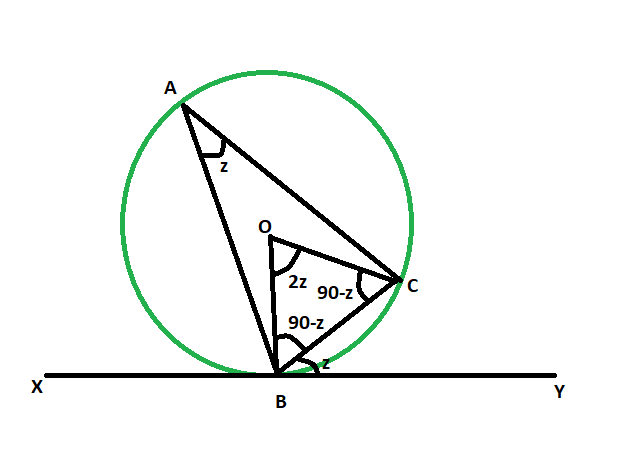# Angle between a chord and a tangent when angle in the alternate segment is given

Given a circle whose chord and tangent meet at a particular point. The angle in the alternate segment is given. The task here is to find the angle between the chord and the tangent.

Examples:

```Input: z = 48
Output: 48 degrees

Input: z = 64
Output: 64 degrees
```Approach:

• Let, angle BAC is the given angle in the alternate segment.
• let, the angle between the chord and circle = angle CBY = z
• as line drawn from center on the tangent is perpendicular,
• so, angle OBC = 90-z
• as, OB = OC = radius of the circle
• so, angle OCB = 90-z
• now, in triangle OBC,
angle OBC + angle OCB + angle BOC = 180
angle BOC = 180 – (90-z) – (90-z)
angle BOC = 2z
• as angle at the circumference of a circle is half the angle at the centre subtended by the same arc,
so, angle BAC = z
• hence, angle BAC = angle CBYBelow is the implementation of the above approach:

## C++

 `// C++ program to find the angle ` `// between a chord and a tangent ` `// when angle in the alternate segment is given ` ` `  `#include ` `using` `namespace` `std; ` ` `  `void` `anglechordtang(``int` `z) ` `{ ` `    ``cout << ``"The angle between tangent"` `         ``<< ``" and the chord is "` `         ``<< z << ``" degrees"` `<< endl; ` `} ` ` `  `// Driver code ` `int` `main() ` `{ ` `    ``int` `z = 48; ` `    ``anglechordtang(z); ` `    ``return` `0; ` `} `

## Java

 `// Java program to find the angle ` `// between a chord and a tangent ` `// when angle in the alternate segment is given ` `import` `java.io.*; ` ` `  `class` `GFG  ` `{ ` ` `  `    ``static` `void` `anglechordtang(``int` `z) ` `    ``{ ` `        ``System.out.print( ``"The angle between tangent"` `            ``+ ``" and the chord is "` `            ``+ z + ``" degrees"``); ` `    ``} ` `     `  `    ``// Driver code ` `    ``public` `static` `void` `main (String[] args)  ` `    ``{ ` `        ``int` `z = ``48``; ` `        ``anglechordtang(z); ` `    ``} ` `} ` ` `  `// This code is contributed by anuj_67.. `

## Python3

 `# Python3 program to find the angle ` `# between a chord and a tangent ` `# when angle in the alternate segment is given ` `def` `anglechordtang(z): ` ` `  `    ``print``(``"The angle between tangent"``,  ` `          ``"and the chord is"``, z , ``"degrees"``); ` ` `  `# Driver code ` `z ``=` `48``; ` `anglechordtang(z); ` ` `  `# This code is contributed ` `# by Princi Singh `

## C#

 `// C# program to find the angle ` `// between a chord and a tangent ` `// when angle in the alternate segment is given ` `using` `System; ` ` `  `class` `GFG  ` `{ ` ` `  `    ``static` `void` `anglechordtang(``int` `z) ` `    ``{ ` `        ``Console.WriteLine( ``"The angle between tangent"` `            ``+ ``" and the chord is "` `            ``+ z + ``" degrees"``); ` `    ``} ` `     `  `    ``// Driver code ` `    ``public` `static` `void` `Main ()  ` `    ``{ ` `        ``int` `z = 48; ` `        ``anglechordtang(z); ` `    ``} ` `} ` ` `  `// This code is contributed by anuj_67.. `

Output:

```The angle between tangent and the chord is 48 degrees
```

GeeksforGeeks has prepared a complete interview preparation course with premium videos, theory, practice problems, TA support and many more features. Please refer Placement 100 for details

My Personal Notes arrow_drop_upCheck out this Author's contributed articles.

If you like GeeksforGeeks and would like to contribute, you can also write an article using contribute.geeksforgeeks.org or mail your article to contribute@geeksforgeeks.org. See your article appearing on the GeeksforGeeks main page and help other Geeks.

Please Improve this article if you find anything incorrect by clicking on the "Improve Article" button below.

Improved By : vt_m, princi singh

Article Tags :
Practice Tags :

Be the First to upvote.

Please write to us at contribute@geeksforgeeks.org to report any issue with the above content.